## 2 August 2017

### Answer key to CEED 2017 Part-A question paper

You are here >> CEED 2017 Part-A solution

I somehow managed time to answer CEED 2016 question paper (exam 1).  Hope you find this post useful. Surprisingly most of the questions covered UCEED syllabus like paragraph comprehension etc. So, like I usually suggest, you may consider practising both CEED  and UCEED previous papers and Mock tests.

### Answers for CEED 2017 PART-A

1) 18

To avoid confusion, just write down the animal names in a rough paper.

tortoise, leopard, mouse, rabbit, dog, crocodile, hen, rhino, monkey, Ram, elephant, horse, snake, Ox, whale, cat, snail, camel

now you see how many you have.

2) 56

As seen i nthe rectangle which has been extruded, we see that since the shape has 4 sides (4 sides of the rectangle), we have 4 extrusions of the wall. So, the key is finding the no. of walls/sides of the given shape. In the below image, I've marked the sides.

Considering the given weird shape, as beIng made of set of six 'Y' shapes with a center solid circle. Now, as shown in the below image, trying the count the number of sides of the shape will result in the following total

No. of Y shapes = 6
No. of sides for each 'Y' shape = 8 (marked from a1 to a8)
No. of extrusion for the six Y shapes = 6*8 = 48
No. of sides for the circular part = 6 (marked from b1 to b6)
Now that we have counted the no. of side extrusions, we are left with a surface at the top and another surface at the bottom, accounting to 2 no's
So, total no. of surfaces after extrusionn = 48 + 6 + 2 = 56

3) 6

In each square, if we multiply the bottom 2 numbers we will end up getting the number given i nthe topb boxes. For ex., consider the first box, bottom multiplied no. = 6*6 = 36, which is given in the top rows of the box. Similarly in the fourth given pattern, 9*4 = 36, so, 6 is the answer.

4) 4

After the cut square portion of 3sm side is removed, all the four regions in the leftover part are of same shape and size. So, the area of the shaded part is 1/4th of the area of the leftover piece.

Actual Area of the square = 5*5 = 25
Area of the cut square of side 3 cm = 3*3 = 9
area of the leftover = 25-9 = 16
Area of the shaded part = 16/4 = 4 cm square centimeters

5) 12

As you can see in the below image, from west we can take any three starting points, marked as R1, R2 and R3. Considering only R1, we have four possible routes as shown by lines in different colors. Similarly, from R2 and R3, we can go in 4 diff routes from each start position. So, total possible rooutes are 4*3 = 12

6) 12

To avoid confusion, I've marked the pentagons on the back side and front side of the 3D solid separately.

Pentagons on the backside

Pentagons on the front side

7) 114

Considering only the bottom most row with al lthe cubes in place, we can say

No. of total cubes = 8 rows X 8 columns = 64 cubes
so, we can say that 32 cubes are white and 32 are blue.

Considering second layer of cubes (from bottom), we observe that 4 cubes (even no.) are missing, so considering 2 as white and 2 as blue, we have the total no. of blue cubes = (32 - 2) = 30 cubes

Considering the third layer of cubes (from the bottom), we observe that 5 cubes (odd no.) are missing and since white cubes are visible at the edges, we can deduce that more no. of blue cubes are missing,  so, we take 3 blue cubes out of 5 missing cubes. So, total no of blue cubes in the third layer = 32- 3 = 29

Considering the top layer (fourth layer), 16 cubes are missing, and again since white cubes are more visible at the edges, we consider more no. (16/2+1=9) of blue cubes missing, so  blue cubes missing and so, no. of blue cubes available = 32 - 9 = 23

Finally, total no. of blue cubes in the structure = 32 + 30 + 29 + 23 = 114

8) 39

Considering only one of the extended part, and as is clear from the below picture

No. of surfaces for each extra part = 8
No. of surfaces considering three parts = 8*3 = 24

If you're confused on the surfaces numbered 5 and 6 in the above picture, below picture might help you. Those surfaces are visible in different view.

Now, considering the central cylindrical cut part. As shown in the below image, you can visualize the top of the shape as being one flat surface marked as one in the below picture. Note that it is continuous as no breaks are visible.

Now, consider the bottom view of the above image, will look like the below image, try to identify the surfaces made by the central cut cylindircal portion. You may not be able to view all the surfaces as numbered by me in the image but just remember that the pattern formed by surfaces 1,2,3,4 (which are cleearly visible) repeat itself two more times (5,6,7,8) and finally (9,10,11,12).

The outer surface is again continuous and hence to be count as one surface, like wise inner surface adds to one surface

Total no. of surface = 24+1+12+2 = 39

9) 3

Thanks to Bhaskar Komara for sharing with us the detailed answer. He has out effort in making this.

Basically, the question is asking about the number of surfaces of the solid formed by the intersection of the given set of solids.

Additionally, check the below image for finding the number of surfaces

I have shown the no. of surfaces in the front direction only which will be 5.
Since the shape is symmetry, no. of surfaces from the backside = 5

No. of surfaces viewed from right side (considering cylindrical surface) = 5
No. of surfaces viewed from left side =  3
No. of surfaces in top view= 1
No. of surfaces in bottom view = 3

Total No. of surfaces = 5+5+5+3+1+3 = 22

10) 250.996

Length LR = Length MR = Radius of the  circle = 42/2 = 21
Length MN = Half of the circumference of the circle = 2*PI*r/2 = PI*r = (22/7)*21 = 66
Length NO = Half circumference - Length of the arc OQ = PI*r - r*theta = 66 -21*(30*PI/180) = 55.004 (approx)

note that 30 degree angle been convereted into radian which will be 30*PI/180 since 2*PI radians makes 360 degrees.

Similarly,
Length OP = Half circumference + Length of arc OT = 66 + r*theta = 66 + 21*(60*PI/180) = 87.992 (approx)

So, total length = 21 + 21 + 66 + 55.004 + 87.992 = 250.996

11) A,D

I'm arraging the given relations like following

Bulls > snails (1)
cats < bulls  (2)
snails > dogs (3)
dogs < bulls (4)
cats > snails (5)

arraning them in ascending order
bulls > cats > snails > dogs

A. cats move faster than bulls is false since it is opposite to (2)
B. Dogs move slower than cats  is true according to the above arrangment. Infact dogas are the slowest of all sccording to all the statement
C. Bulls move faster than dogs is false according to (4)
D. Bulls move slower than cats is false according to the arrangement above

12) A,C

It's obvious that all the given part logo's belong to Indian banks. So, A, B and C suits. But observe that the SBI logo given in the option is not correct since it should have a circle in the middle and not rhombus. A being canara bank logo and C being the logo of HDFC bank, so they are the required options.

13) B,C

Blind spot is a particular part of human eye and is nnotassociated with color perception.

An afterimage is an image that continues to appear in one's vision after the exposure to the original image has ceased. This is nothing but an illusionary view on our eyes after prolonged view of something.

INter-ocular distance is the distance between the centers of rotation of the eyeballs of an individual or between the oculars of optical instruments, which has nothing to do with color perception.

14)  A,B,C,D

All the shapes can be obtained

15) A,C

Check this on how to visualize solids -

16) A, B, D

A cantilever is an arrangement of the metal (say a bar/rod etc) such that it overhangs as shown in the below picture. I've drawn an image showing how A can be tilted such that there are no overhanging elements. You cna visualize similarly and check for B,C,D. Note that C has some sort of cantilever form in all the tilt positions.

17) A,B,C
by observation

18) B, C

All the options has al lthe shapes given in the cut shape, but the key is to find the right size and shape of the cut pieces. For ex., If you closely observe otion A and B, both looks same, but part no. 6 is smaller in size in option A.

I've shown the image for option B, try for option C also and see if you can spot the pieces.

More resources and guide for 'Tangram puzzles' are avilable in CEED/UCEED resource page - 1.

Some online sites for similar questions

19) B, D

20) A,B,D

Manthan, Bhumika, Nishant  - Shyam Benegal
Droh Kaal - Govind Nihalani

(I hate this kind of general questions :P, Don't know how this going to help in design :P)

21) D

As shown in the below figure, I used the base landing profile to figure out the shape of 'a' that is of same font kind as the given word. Observe the 'V' kind of landing and the one that is marked in green arrow. Only option D matches.

22) B

(Just took from key, I'm not sure who told this :D)

23) B

Observe the pattern that I showed in red boxes. As you can see they repeat the pattern everywhere the given set of symbols. Based on that, we note that empty triangles are positioned above the dark filled triangle, which is again above the dark filled squasre. Shoe, the two question marks highlighted by blue rectangle should have two empty triangles. Only option B has that

24) A

As shown in the below image, If the ant starts at the top (Point X) and wraps around and reaches the point Y (which is vertically below X), and if we assume that we cut the cylinder along XY line and unfold it, we will see that the unfolded cylinder will becoma a rectangle and the path of thr ant will be along the diagonal straight line conencting A and B, as shown in figure.

Length of OB = circumference of circle of diameter 5 cm  = 2*PI*R = PI*D = PI*5

According to pythogorous theorem,
Length of AB = square root of(AO square + OB square)
= square root of(5 square + PI*5 square) = square root of(25 + 25*PI^2)

25) D

Given CAT ~ BES

observe each alphabet in both sides, (C,B) (A,E) and (T,S)
C comes next to B, and T comes next to S
Also, we know that vowels are (A,E,I,O,U) so the pair (A,E) is the consequtive vowle alphabets in the list of vowels

now for the second coondition,

DOG ~ CUF
observe each alphabet in both sides, (D,C) (O,U) and (G,F)
D comes next to B, and G comes next to F
Also, we know that vowels are (A,E,I,O,U) so the pair (O,U) is the consequtive vowel alphabets in the list of vowels

SO KIN ~ ?
K comes next to J, so the first alphabet should be J, similarly N comes next to M, so the last alphabet is M. The middle alphabet is given as I, in the vowel list a,e,i,o,u, O is the next alphabet to I,
So, the required word is
JOM

26) B

27) C
Most of the regularr/general used engines (like TVS, Hero, Bajaj) have single cyclinder. Only big and heavy motorcycles that needs large power/torque (including race bikes) need multiple cylinders.

28) A

For more about the other symbols, check this pdf

29) C

As you can see in the below image, View Y is obtained when you rotate about the shown axis, around 180 degrees. So, the following has to be observed
1. Back face of view X is the front face of view Y
2. front face of view X is the back face of view Y
3. Right face of view X is the left face of view Y
4. Left face of view X is the right face of view Y

So, as shown by arrows, the square and the rectangle shapes on the right face of the view X, should be on the invisible surface (eft side) of view Y.

Now If you closely observe view Y and Z, the semi circle on the front face of Z is just flipped as that seen in Y. So, it means that Z is the rotated view of the Y (180 degrees) as shown in figure. So, the left side of View Y is the right side of the view Z, but note that the upper part of left Y will now be at the lower section of the right Z. This is shown with dotted line arrows.

30) B

For paper fold and cutting kind of questions, the best way is to move in reverse order - I mean by way of unfolding and mirroring the cut pattern along the line of fold/unfold. Note that unfolding is just the reverse of how it was folded, which you can compare the compare in the question and what I drew below.

31) A

List of Thomas A Edison's inventions - Phonograph, The Electrographic Vote Recorder, Motion Picture device, Magnetic Iron Ore Separator, many patents related to Telephonic telegraphs,

Kirkpatrick Macmillan is with inventing the pedal driven bicycle.

Elisha Graves Otis was an American industrialist, founder of the Otis Elevator Company, and inventor of a safety device that prevents elevators from falling if the hoisting cable fails.

George Eastman was an American entrepreneur who founded the Eastman Kodak Company and popularized the use of roll film, helping to bring photography to the mainstream. Roll film was also the basis for the invention of motion picture film in 1888 by the world's first film-makers Eadweard Muybridge and Louis Le Prince

Source: wikipedia

32) C

Height of the wall = 10 feet

first attempt
Distance travelled by the green lizard = 3 - 1 = 2 feet
whereas, distance travelled by the red lizard = 4 -2 = 2 feet

So, in each attempt, both will have climbed the same distance.

fourth attempt
height touched by the green lizard = height reached by previous three attempts + maximu height it can reach = 2*3 + 3 =9,
although the green lizard has tried to move 3 feet, since it has not reached the top, it will slip by 1 feet, and so the net height reached by it is 9-1 = 8feet

In fourth attempt of red lizard,
height touched by the red lizard = height reached by previous three attempts + maximum height it can reach = 2*3 + 4 =10,

So, in the fourth attempt, it can reach the top of the wall and so there is no notion of slipping.

33) B

This is similar to tessellation problem. The best way is to find this is: at each step, try folding each surface to form back a cube and see in each folding, whether the red line is the folded surface is in line (at the same point) with the red line of the surface to which the current folded surfaces closes.

As an example, check the below image for option A, and notice that keeping surface 2 on the floor, if I try to fold surface 1 and surface 3, both will stand up right making contact at the edge denoted as 'a' circled. So, for the line to be continuous, the distance d1, and d2 should be equal, otherwise both won't be along the same point of the contact edge. Hope you got this. In option A, as you can see, it is straight forward that d1 is not equal to d2, and so we conclude that it is not correct.

While checking the other options, instead of finding for correct match, try to find a MIS-MATCH, which will save your time for solving. Hope you understood this.

34) A

As can be seen in fig (a) below, if North is mistaken as being in south east direction, then the rest of the major directions will be as shown in the figure (a).

As per their given statement, if I position school and play grround 100 meter each along east and south directions, then the playground end up in straight vertical upwards from school as shwon in fig (b)

35)  A

Note that the links DH, DG, OD, OB, BG, are all equal. Assume that ends A and C are moved simultaenously with equal angles. If A is held and turned anti clockwise direction, then it is dragginb B to the left, which again drags G to the left. Similarly if we hold C and turn it clockwise direction as shown in the question, then we are trying to drag D to the left (just like A did with B), which again tries to drag G to the left. So, assuming equal movements of A and C, and since lengths are equal, it is clear that G tends to be dragged towards O, alongg the horizontal line joining both. Similarly H tends to move down along the vertical line through O.

36) A

More about colours can be found on this resource page

37) B

You observation should be on the thumb finger first to help in recognizing the mistake quickly. If the yellow guy is standing to the left and is hifying with his right hand, then then thumb should be on the left to his hand, right ? Thus, option C is out from the list.
The blue guy is facing him and is hifying with his right hand, so according to his standing position, his thumb should be to the right according to what we view. So, only option B suits.

38) C

This is very straight forward observation question, in each step, the pattern gets rotated clockwise to 45 degrees as is clear in the below picture. To understand this just take one square out of the five square parts in the first pattern, and start how it is moving in the next two steps.

39) C

Vincent Vangogh - was a Dutch Post-Impressionist painter who is among the most famous and influential figures in the history of Western art. In just over a decade he created about 2,100 artworks, including around 860 oil paintings, They include landscapes, still lifes, portraits and self-portraits, and are characterised by bold colours and dramatic, impulsive and expressive brushwork that contributed to the foundations of modern art.

Paul Gauguin was a French post-Impressionist artist. Underappreciated until after his death, Gauguin is now recognized for his experimental use of color and Synthetist style that were distinctly different from Impressionism. His work was influential to the French avant-garde and many modern artists, such as Pablo Picasso and Henri Matisse. He was an important figure in the Symbolist movement as a painter, sculptor, printmaker, ceramist, and writer. His bold experimentation with color led directly to the Synthetist style of modern art, while his expression of the inherent meaning of the subjects in his paintings, under the influence of the cloisonnist style, paved the way to Primitivism and the return to the pastoral. He was also an influential proponent of wood engraving and woodcuts as art forms.

Rembrandt was a Dutch draughtsman, painter, and printmaker. An innovative and prolific master in three media, he is generally considered one of the greatest visual artists in the history of art and the most important in Dutch art history. Rembrandt's works depict a wide range of style and subject matter, from portraits and self-portraits to landscapes, genre scenes, allegorical and historical scenes, biblical and mythological themes as well as animal studies. His contributions to art came in a period of great wealth and cultural achievement that historians call the Dutch Golden Age. Rembrandt's portraits of his contemporaries, self-portraits and illustrations of scenes from the Bible are regarded as his greatest creative triumphs. Rembrandt's foremost contribution in the history of printmaking was his transformation of the etching process from a relatively new reproductive technique into a true art form, along with Jacques Callot.

40) A

Arranging R n top of P, and by careful observation and comparison iwth S, we can deduce that A is the required pattern.

41) C

There are usually 24 frames per second in a film. So, for a min, the number of frames would be 24*60 = 1440. Goven that in one minute, it took 90 feet projection of the film.
90 feet projection -> 1 min -> 1440 frames
90 feet projection -> 1440 frames

So, dividing both sides by 90,
1 foot projection -> 1440/90 frames
which is 16 frames

In 1 min, 90 feet of film is projected,

42) A

Fitts's law is a predictive model of human movement primarily used in human–computer interaction and ergonomics. This scientific law predicts that the time required to rapidly move to a target area is a function of the ratio between the distance to the target and the width of the target.

So, according to this law, for lesser time requirement, the distance to the target should be less and the width of the target area should be more. In the module X, when we press the letter 'Sa' in hindi, the circular menu pop up which was arranged such that all the variants of that letter are near to the center and the width/space occupied by them is more, compared to the configuration shown in Y, in which some of the letters are far from the initial touch position, and the width/space occupied by the variants are very less.
So ,time when operaton module X will be comparitively less.

43) A

When the ball is released fr omthe top, it rolls, reaches P and then due to inertia and the potential energy saved in it, it tires to continue along the curve, but the energy it has in storage is not sufficient to make it cross the contour, and hence the ball tend to osciallte along the curve on both sides until it comes to a halt at P.

44) B

If you turn the given word 180 degrees, then you will see the same word.

Palindrome - A palindrome is a word, phrase, number, or other sequence of characters which reads the same backward as forward, such as madam or racecar.

An ambigram is a word, art form or other symbolic representation whose elements retain meaning when viewed or interpreted from a different direction,  direction, perspective, or orientation.

Example images of Ambigram

I'm not sure about the rest two names, I think they picked the names randomly.

45) A

Since subject A appears to the right, the camera which is placed over-the-shoulder should be to the left relative to the person (A). So, camera 2 is the required. Similarly since subject B needed to be to the left, the camer should be right to B, so, camera 3 suits the requirement.

1.Bhanu the answer for the 9th question in the final answer key uploaded in the ceed site is 3 but here you have mentioned it as 22. Can you help me understand .

1.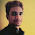I rechecked the ques, they said, "how many surfaces will the resultant solid have?" While i counted the surfaces of the overall solid formed, I think they meant the surface count of the only solid portion, which represent the union of three. I will try to draw it and will check if that makes a count of 3. Will update soon. Thanks Riya.

2.Answer for Q12 is given as A,C.

3.oops, my bad, I didn't notice the canara bank logo and I neglected the fact that SBI logo is not correct, it should have circle in the center, which is not shown in the image. Thanks for spotting that.

4.question says 'INTERSECTION' (common portion of the three)

5.9th Question.. the new solid formed by INTERSECTION have 3 surface only.. not 22 which is union of solids

6.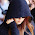7.2.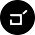sir, Q16 , where is the diagram that u hav drawn

1.yeah, thanks, just noted, updated, thanks for informing

3.answer 18 should be B and C i guess

1.In C, the square shape is much bigger in size than the given rectangle in the ques, so it can't be. Compare its size with that of the biggest triangle so that it will be easy for u to spot the diff.

2.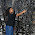yes exactly, in C option, the size of the square is bigger than the image given in question. that's why the answer should be B and C instead of D.. as the D option is okey.

3.yes! answer should be B and C, Square is bigger than the actual figure

4.Yeah, my mistake, I've changed it

4.sir plz explain Q 40

1.Although, it's bit difficult to explain in words, let me try to explain.

1. Assume P, Q, R and S as plain papers with cuts/holes as shown in the question (except Q) - Or visualize them as stencils
2. Place paper P on top of paper R (or vice versa) and visualize the overlapped zone. From this, u can get the cut portion visible. I mean the portion of thee overlapped papers which are cut in both the papers
3. Now, if we overlap paper Q (with unknown cut patterns) over the combo of P and Q, we should see the cuts as in S. So, visualize and see from the given options A,B,C,D which one suits the need.

Although this appears like we need papers physically, but in actual, you can visualize them mentally to observe the required pattern. Like if u observe the cuts in the top left corner of both P and Q and if they have to be overlapped, some are of the uncut portions of P on top left corner covers the cut portion of top left corner of R, so the combination will have a reduced cut portion. Something like this.
Give it a try.

5.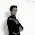you are doing great job Bhanu. All those answers were elaborated very well and are helpful..

1.Thank you brother

6.kindly change the value of ans.10(152) the value should be 250.996. if the ans is ok then kindly tell me the whole procedure i am unable to get it.

1.I've already given the explanation and in that u can see 250.996, but in the answer, i made mistake in typing. Procedure is same like i showed in the pic.

2.Sir the pi value is said to be 22/7 so the decimal value wont come in the ans

7.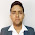12) A,C

It's obvious that all the given part logo's belong to Indian banks. So, A, B and C suits. But observe that the SBI logo given in the option is not correct since it should have a circle in the middle and not rhombus. B being canar bank logo and C being the logo of HDFC bank, so they are the required options.

sir in this ans in the line 3rd you miss typed (B being canar bank). It must be typed as (C being canara bank).

Kindly change it sir it creating a lot confusion.

1.Thanks for pointing out, but actually A is canara bank logo and not C.
And btw no need to get confused for small things like this ques :) you could just google it and clarify if u felt doubt. And I might not have given correct answers. Mistakes happen, that's why even official answer key is been corrected at least once.

2.ok sir :)

8.Dear sir,

ans. 18) Options must be B & C.

1.yeah, my bad. That's my mistake, I think half-understood the ques :|
I corrected that.

9.Sir, in question 9, surfaces will be like plan=7, front and back will be 5+5, sides will be= 5+5, below will be= 5 , so the total surfaces should be 7+5+5+5+5+5= 32

1.I think u r recounting the same cylindrical surface in multiple views. I suggest u to chk my video guide on how to deal with this.

2.or am i missing something here? the question says - find the no. of surfaces for the intersection. if we consider the intersection of three given solids removing the non coincident solids, then that shld count to 3! The solid as a whole add upto to 22 surfaces

10.Q15 why D is not an ans..??!

1.i think as in D the figure show dotted line i.e there must be a groove but in Fig A we can see it is solid or else as we have seen from top and front we might have seen the depth .

11.sir if there is two correct answer so how to choose right one because any student will choose one option at a time.

1.ceed has MSQ type too - multiple selection, I suppose u better chk the sectionwise pattern of ceed!

12.Sir, In q.10 how did you calculate the angles as 30 and 60 degree. As it is not given in the question. Kindly explain.

13.Ok, i got this 😃

14.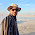sir can u answer ceed 2017 part b, the second last question which is based on symmetry, what is the solution and how to solve it?

1.harsh,
ceed 2017 pat b doesn't seem to have the ques u mentioned! pls chk

15.Sorry ,My mistake, its M.DES 2017 of NID and question number 70.
Please suggest solution or else the approach.

1.np, will chk that soon n update if i can answer

16.QSTN 15, WHAT ABOUT OPT.D SIR ?

1.D is not form knot,but b is also answer,plz check it sir

2.D is not form knot,but b is also answer,plz check it sir

3.it's given that front n top views r identical, so the small inner square in the top n side view measures same side length. But in option D, it's not equal which can clearly be seen by dashed lines. That's the reason why B is also not considered, In fact D is almost similar to D except that D has holes, and B has projections(extrusions), the size of the squares being dissimilar.

17.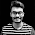Bhanu sir, can you please upload the question paper with answer sheet for 2016 chennai region examination. thankyou.

1.will try soon.

18.Bhanu sir, i guess your explanation for Q27 is not correct. every Enfield bike manufactured till date has only single cylinder/piston. the reason for upper 2 half's design of engine is due to the inlet and outlet valves.

19.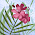In question 14 how is hexagon obtained?

1.consider any two opposite corners diagonals (one at top and the other at opp. bottom corner) and instead of imagining a plane through the diagonal corners, move the plane a little such the cut plane at the top surface has some length, similar to the cut face at the bottom. You will get a hexagon!

20.In question 16 how is option D?

21.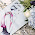Sir question 15 ,why not option D,since there is a square cross sectional cut out

1.it's given that front n top views r identical, so the small inner square in the top n side view measures same side length. But in option D, it's not equal which can clearly be seen by dashed lines. That's the reason why B is also not considered, In fact D is almost similar to D except that D has holes, and B has projections(extrusions), the size of the squares being dissimilar.

22.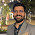Hi Bhanu, Question 1 -In this question, we have to identify unique shapes. You have written tortoise in your answer which is not a unique shape. My answer is coming out to be 9 and your answer is 18. Please check and confirm.
Thank you

1.u haven't got the ques properly it seems, the ques is abt finding all the shapes which are diff from other, but not repeated.

23.sir could you explain Q16 a little more clearly

24.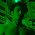Sir could you explain the Q 16 a little bit clearly pls

25.Sir, could you please sketch and show how the cross scetions in Q.14. could be pentagon and hexagon?

26.In question 16, looks like option D does have a cantilever in all positions. Can you please explain where it might not?

27.28.Dear Sir, If you get time please tell me in question 10) how you are getting the angle to be 30 AND 60. It could have taken any value? or 60-30

29.Sir, in the 5 th question it is mentioned without retracing. But if we choose first outlet in 4 different way , we are also retracing 2 ways ( after the intersection in the middle). Also, why we are not considing the 3rd outlet?

30.Bro won't questions 16 have only a,b as answer

1.D can be arranged such that there is no cantilever, it's possible, check. Arrange it such that it assumes the legs of a chair.

31.sir plz help me with q.14

32.In Q10, Could you please explain how you calculated the 30 and 60 degrees ?

1.the length of the blue line shown is 2*radius and the end of it (point S) is lying horizontally along the tangent of the circle horizontally (notice the red arrow) So, it's height is r = radius,
with the known details: hypotenuse = 2*r, and opposite side = r, you can get the angle of the triangle, using which it is easy to find 30 degree. 60 deg on the other side because, in geometry, it's angle will be 90-30 = 60.

Your comment will be sent to Bhanu for his approval. It will get published after his approval. You will receive mail for all the replies to your comment.